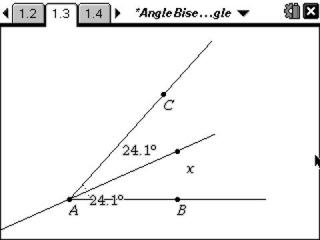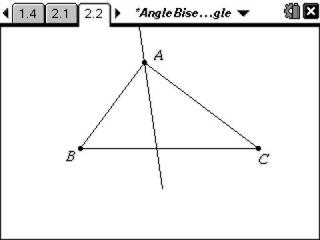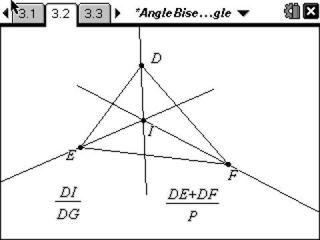# Activities

••• ##### Subject Area

• Math: Geometry: Triangles

• ##### Author9-12

40 Minutes

• ##### Device
• TI-Nspire™
• TI-Nspire™ CAS
• ##### Software

TI-Nspire™
TI-Nspire™ CAS

2.0

• ##### Other Materials
By Karen Campe

## Angle Bisectors in a Triangle#### Activity Overview

In this activity, students will explore the relationships between an angle bisector and segments in a triangle. They will determine the distances from an angle bisector to the sides of the bisected angle. In a triangle, proportional relationships occur when an angle bisector divides the opposite side into two parts.

#### Key Steps

•First, students will investigate the Angle Bisector Theorem. Students should see that the angle bisector is equidistant from both sides of the angle.

•Next, students will explore an angle bisector in a triangle. Students should see the relationships between the sides of the triangle and the bisector.

•As an extension, students will explore the incenter of a triangle.